# Sets - Examples (with Solutions), Algebra, Quantitative Aptitude Part 1 Notes | Study Quantitative Aptitude for GMAT - GMAT

## GMAT: Sets - Examples (with Solutions), Algebra, Quantitative Aptitude Part 1 Notes | Study Quantitative Aptitude for GMAT - GMAT

The document Sets - Examples (with Solutions), Algebra, Quantitative Aptitude Part 1 Notes | Study Quantitative Aptitude for GMAT - GMAT is a part of the GMAT Course Quantitative Aptitude for GMAT.
All you need of GMAT at this link: GMAT

Defining a Set

"Set" is synonymous with the words "collection", "aggregate", "class" and is comprised of
elements/objects/members.

A set is a well-defined collection of elements. By well-defined elements it means
that given a set and an element, it must be possible to decide whether or not the
element belongs to the set. A set can be described in any one of the following
ways. For example, the set of beautiful Actress of Bollywood or the set of Good
Players of cricket in India are not sets as the world beautiful and Good are not well
defined. What are the criteria of choosing an actress as beautiful and which player
is said to be a good player of cricket. But on the other hand if we say Set of good
player of cricket in India those has played at least 25 international games, will be a
set as the word Good is now well defined.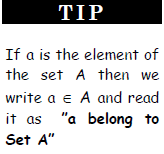Representation of a Set

A set can be described in two different ways.

1. Roaster Form:
A set is described by listing elements, separated by commas, within
brackets. For example, A = {a, e, i, o, u} is a set of vowels of English
alphabets and a finite set and N = {2, 4, 6,…} is a set of even natural
number and is a infinite set. Also, repetition of an element has no effect.
For example {1, 2, 3, 2} is the same set as {1, 2, 3}.

2. Set Builder Form:
A set can also be described by a characterizing property P(x) of its
elements x. In such a case the set is described by {x | P (x) holds} or {x :
P(x) holds}, which is read as 'the set of all x such that P(x) holds'. For
example,
A = {x | x is the root of x2 + 5x - 6 = 0}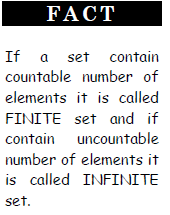Cardinal number of a set

The number of elements contained in a set is known as the cardinal number of that set. On the basis of
cardinal number a set can be an Empty Set or a Singleton Set. A set is said to be empty or void or null set if
it has no elements and its cardinal number is 0. It is denoted by the symbol φ or { } such as Set of odd
numbers divisible by 2. And a set is singleton set if the cardinal number is 1 i.e. it contains only one element,
such as Set of lady Prime-minister of India.

If a set A has 5 elements then its cardinal number is written as n(A) = 5, and read
as “Cardinal number of set A is 5”.

Subsets
Let A and B be two sets. If every element of A is contained in B, then A is called
the subset of B.

If A is subset of B, we write A ⊂ B, which is read as "A is a subset of B" or "A is
contained in B".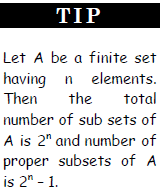If A ⊂ B then a ∈ A ⇒ a ∈ B. (The symbol ⇒ stands for "implies" and ∈ stands for “belongs to”).
Let P = {a, b, c}, then subsets of P are φ, {a}, {b}, {c}, {a, b}, {a, c}, {b, c}, {a, b, c} i.e. it has 8 number of
subsets and Empty set is the subset of every. Also every set is the subset of itself and it is improper subset of the set. Every set has one improper subset and other subsets as proper subsets.

If A ⊂ B and also B ⊂ A, then we can say every element of A is present in B and every element of B is
present in A. These types of sets having same number and identical elements are known as Equal sets.

Power Set

The set of subsets of a set is the power set of the set. If A is a set then its Power set is denoted as P(A).
If A = {a, b, c}, then P(A) = {φ, {a}, {b}, {c}, {a, b}, {a, c}, {b, c}, {a, b, c}}

The cardinal number of any set A is 2n, where n = number of elements present in set A.

Universal Set
In any discussion in theory, there happens to be a set U that contains all sets under consideration. Such a set
is called the universal set. Thus a set that contains all sets in a given context is called the universal set. For
example, in plane geometry the set of all points in the plane is the universal set.

Some Important Universal Sets:
N = Set of all natural numbers = {1, 2, 3, 4,…}
Z or I Set of all integers = {…- 3, - 2, - 1, 0, 1, 2, 3,..}
Z+ = Set of all positive integers = {1, 2, 3,…} = N
Z- = Set of all negative integers = {- 1, - 2, - 3,..}
W = Set of all whole numbers = {0, 1, 2, 3,…}
Z0 = The set of all non-zero integers = {± 1, ± 2, ± 3,..}

Q = The set of all rational numbers.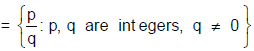R = The set of all real numbers.

R - Q = The set of all irrational numbers.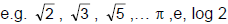etc. are all irrational numbers.

Operations of sets
Union of Sets:

The union of two sets A and B, is a set containing all the elements present in set
A or in set B. The union of set A and B is represented as A U B.
So, AUB = {x | x ∈ A or x ∈ B}

For example:
A = {5, 7, 8, 9, 11} and B = {car, house, ball, sofa), then
A U B = {5, 7, 8, 9, 11, car, house, ball, sofa}

Intersection of Sets:
The intersection of two sets A and B, is a set containing all the common
elements present in set A or in set B. The intersection of set A and B is represented as A ∩ B.
So, A ∩ B = {x | x ∈ A and x ∈ B}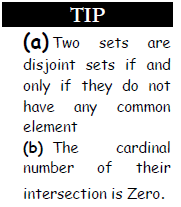For example
A = {5, 7, 8, 9, 11} and B = {3, 4, 5, 6, 7, 8}, then
A ∩ B = {5, 7, 8}

Difference of sets:
A - B is the difference of set A from set B and is defined as the set of elements
present in set A but not in set B.
So, A - B = {x | x ∈ A and x ∉ B}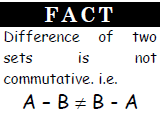Complement of a set:
The complement of set A in U the set of those elements which are present in Universal set but not present in
set A. Compliment of A is denoted by A' The shaded part represents A'.
So, A‘= {x | x ∈ U and x ∉ A}

(a) Also the Compliment of set A is defined as the difference of Universal set U and set A. i.e.⇒ A‘ = U - A

Facts and Rules:

i. A ∪ A = A & A ∩ A = A

ii. A ∪ φ = A & A ∩ φ = φ

iii. A ∪ U = U & A ∩ U = A

iv. A ∪ B = B ∪ A & A ∩ B = B ∩ A

v. A ∪ A’ = U & A ∩ A’ = φ

vi. n(A ∪ B) = n(A) + n(B) - n(A ∩ B)

vii. n(A ∪ B ∪ C) = n(A) + n(B) + n(C) - n(A ∩ B) - n(B ∩ C) - (C ∩ A) + n(A∩B∩C)

viii. n (A - B) = n (A) - n (A ∩ B)

Sol. Let A & B denote sets of people who read paper A & paper B respectively and in all there are 100
people, then n(A) = 25, n(B) = 20, n (A ∩ B) = 8.
Hence the people who read paper A only i.e. n(A - B) = n (A) - n(A ∩ B) = 25 - 8 = 17.
And the people who read paper B only i.e. n(B - A) = n (B) - n (A ∩ B) = 20 - 8 = 12.
= [(30% of 17) + (40% of + 12) + (50% of 8)]% = 13.9 %.

Venn Diagrams

Venn diagram is the pictorial representation of the set and also the operation involved in the sets. We often
use circles to represent the sets and overlapping of the circles to represent the common elements in two or
more sets. The universal set U is represented by interior of a rectangle and its subsets are represented by
interior of circles within the rectangle. Below are some examples of Venn-diagrams.

1. U = universal set
A = subset of U
A’ = complement of A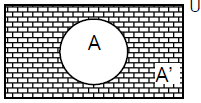2. The representation of A U B in Venn diagram is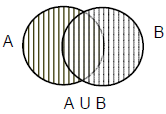3. The representation of A ∩ B in Venn diagram is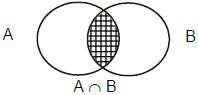4. Difference of set A from B i.e. A - B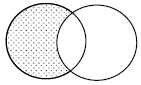Maximum and Minimum elements in a set

Let us consider the formula, n(A ∪ B) = n(A) + n(B) - n(A ∩ B) and imagine that the value of n(A ∪ B) is not given and asked to find the value of n(A ∩ B), then we will get the range of the values. Let us understand the concept of maximum and minimum values with the help of an example.

Ex.2 In a B - school there are three specializations in Management course and a student is free to specialize in any number of fields. These specializations are Finance, Marketing and HRD. If 120 students specialize in Finance, 110 in Marketing and 125 are in HRD. 90 students have finance and Marketing both as their specialization, 85 have marketing and HRD while 80 have both finance and HRD. What can be the minimum and maximum number of students who can specialization in all the three fields?

Sol. Let us consider that the number of student who enroll in all the three streams be x. Thus according to the Venn diagram drawn with the help of given information.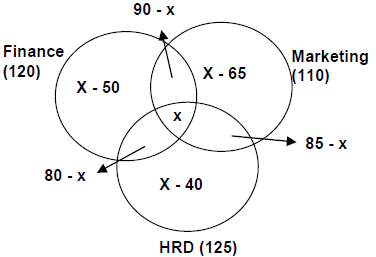Now for the minimum value of x, we have x - 65 ≥ 0 ⇒ x ≥ 65
And for maximum value of x, we have 80 - x ≥ 0 ⇒ x ≤ 80
Hence 65 ≤ x ≤ 80. So we have the range of the values that can be the required solution.

Ex.3 If in a Survey, Organized by any N.G.O on the cold drinks after effects found that 80% of the total people like Coca - Cola and 70% like Limca. What can be the minimum and maximum number of people who drink both the drinks?

Sol.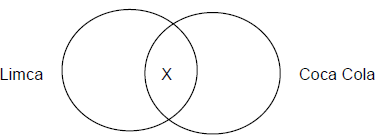Here n(L ∪ C) ≤ 100%
Using the formula, n(L) + n(C) - n(L ∩ C) = n(L ∪ C) ≤ 100%
80 + 70 - X ≤ 100
Solving we get X ≥ 50%, Also X ≤ 70%
Minimum value = 50% and Maximum value = 70%.

The document Sets - Examples (with Solutions), Algebra, Quantitative Aptitude Part 1 Notes | Study Quantitative Aptitude for GMAT - GMAT is a part of the GMAT Course Quantitative Aptitude for GMAT.
All you need of GMAT at this link: GMATUse Code STAYHOME200 and get INR 200 additional OFF

## Quantitative Aptitude for GMAT

75 videos|87 docs|156 tests

Track your progress, build streaks, highlight & save important lessons and more!

,

,

,

,

,

,

,

,

,

,

,

,

,

,

,

,

,

,

,

,

,

,

,

,

,

,

,

;